Fundamental Theorem of Algebra MathBitsNotebook.com Terms of Use   Contact Person: Donna RobertsIf you know that a quadratic equation (degree 2) has exactly 2 roots, then you already know
(and have used) the Fundamental Theorem of Algebra.

While there are various statements of this theorem,
the interpretation that we are interested in for this course is the following: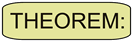The Fundamental Theorem of Algebra: If P(x) is a polynomial of degree n ≥ 1, then P(x) = 0 has exactly n roots, including multiple and complex roots.

In plain English, this theorem says that the degree of a polynomial equation tells you how many roots the equation will have.

A linear equation (degree 1) will have one root.
A quadratic equation (degree 2) will have two roots.
A cubic equation (degree 3) will have three roots.
An nth degree polynomial equation will have n roots.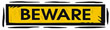Read carefully to fully understand what this theorem is saying.
• It mentions "multiple" roots . . . so, if a polynomial has a repeated root, each repetition of the root is counted.
• It mentions "complex" roots . . . so, if a polynomial has a complex root, then the conjugate is also counted since complex roots come in conjugate pairs.
This theorem deals with complex roots, both real and non-real. Remember that all Real Numbers are a subset of the Complex Numbers.Let's verify that the Fundamental Theorem of Algebra holds for quadratic polynomials.

A quadratic polynomial is a second degree polynomial. According to the Fundamental Theorem of Algebra, the quadratic set = 0 has exactly two roots.

As we have seen, factoring a quadratic equation will result in one of three possible situations.
 The quadratic may have 2 distinct real roots. This graph crosses the x-axis in two locations. These graphs may open upward or downward.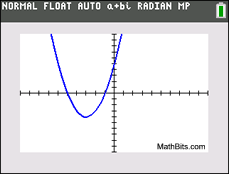It may appear that the quadratic has only one real root. But, it actually has one repeated root. This graph is tangent to the x-axis in one location (touching once).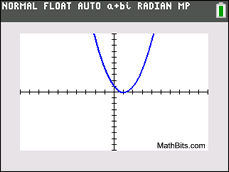The quadratic may have two non-real complex roots called a conjugate pair. This graph will not cross or touch the x-axis, but it will have two roots.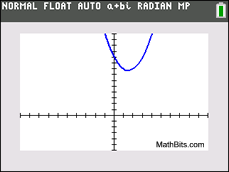In all three situations, there are two solutions to the quadratic equations,
as stipulated by the Fundamental Theorem of Algebra.Historical note: Mathematicians have been unable to devise an exact general method for factoring a polynomial. Factoring techniques (formulas) are known for "special" polynomials such as linear (degree 1), quadratic (degree 2), cubic (degree 3), and quartic (degree 4). Mathematician Evariste Galois (1811-1832) proved that there will never be a general formula to factor polynomials of degree five or higher.